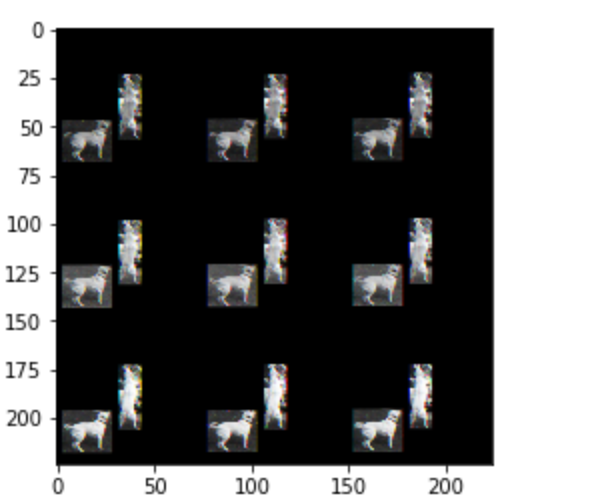# Different activations with same image

``````def get_object_context_features(object_image, context_image):
torch.set_default_dtype(torch.float32)
data = (ImageList.from_folder('/Users/Siddharth/Projects/Wisconsin/causal_discovery/discovering_causal_signaals_in_images/mscoco/dummy/{}'.format('cat'))
.split_none()
.label_from_folder()
.transform(size=224)
.databunch()
.normalize(imagenet_stats)
)
data.train_dl.batch_size = 1
fnames = data.items
feature_extractor = cnn_learner(data, models.resnet18, metrics=[accuracy])
model = feature_extractor.model
feature_extractor.model = nn.Sequential(*(list(model.children())[:-1]), *(list(model.children())[-1][:-4]))
tfms = transforms.Compose([
transforms.Resize(224),
transforms.CenterCrop(224),
transforms.ToTensor(),
#transforms.Normalize(mean=[0.485, 0.456, 0.406], std=[0.229, 0.224, 0.225])
])
object_img_tensor = tfms(PIL.Image.fromarray(object_image))
context_img_tensor = tfms(PIL.Image.fromarray(context_image))
feature_extractor.model.eval()
object_image_features = feature_extractor.model(object_img_tensor[None])
context_image_features = feature_extractor.model(context_img_tensor[None])
return object_image_features, context_image_features
``````

I’m trying to get activations of the pre-final layer(containing 512 neurons) from a pre-trained resnet18 model. The function takes two images as input and these images passed through the feature extraction network mentioned before.

Every time I run this code on the same images, I get different activations. Is this normal? Isn’t this a pre-trained model on ImageNet so shouldn’t the activations be constant on the same image?

try displaying object_img_tensor and context_img_tensor to make sure no random transformation is going on.

Here is the object image before and after applying the transform for one of the images. I dont understand why 9 images show upIt looks like your `feature_extractor` model contains the backbone and piece of the head.

``````feature_extractor.model = nn.Sequential(*(list(model.children())[:-1]), *(list(model.children())[-1][:-4]))
``````

here the `list(model.children())[-1]` refers to the model’s head.
When you call `cnn_learner` the head is created, initialized randomly and attached to the pretrained backbone.

To get the backbone you can simply use `learner.model` right after creating the learner using `cnn_learner`.

Makes sense!
I want to obtain activations from the pre-final layer before the ReLu non linearity is applied so that I get an output of 512 features. How do I get intermediate layer activations like I mentioned above?

The last layer of backbone is a linear layer. Have a look at the model structure printed here. Last layers of the backbone are Conv and BatchNorm:

But the output of backbone is not 512 features. It’s 512 feature maps. Each feature map is a rectangle of some size(depending on the input image size).

I’m sorry, by pre-final layer I meant the Linear layer numbered at 4 in the Head of this model